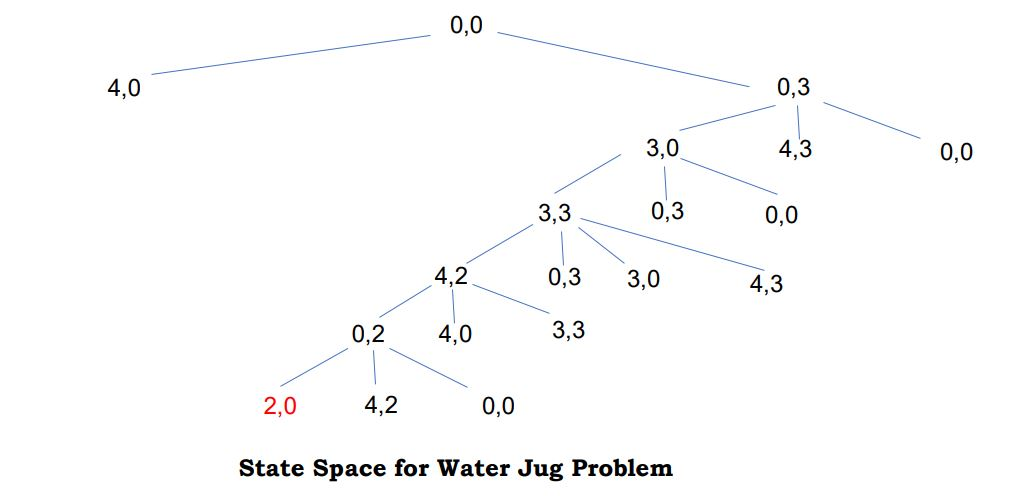# What is state space representation? Illustrate with one example.

The initial state, actions, and transition model together define the state space of the problem implicitly.

State-space of a problem is a set of all states which can be reached from the initial state followed by any sequence of actions.

The state space forms a directed map or graph where nodes are the states, links between the nodes are actions, and the path is a sequence of states connected by the sequence of actions.

Major components of state space representation:

• Initial State
• Goal State
• Operator or legal moves

Example:

WATER JUG PROBLEM

You are given two jugs, a 4-gallon one and a 3-gallon one. Neither has any measuring mark on it. There is a pump that can be used to fill the jugs with water. How can you get exactly 2 gallons of water into the 4-gallon jug?

=>

The state space for this problem can be described as the set of ordered pairs of integers (X, Y)
where,
X represents the quantity of water in the 4-gallon jug X= 0,1,2,3,4
Y represents the quantity of water in the 3-gallon jug Y=0,1,2,3

Then,

Initial State: (0,0)
Goal State: (2,0)
Operator / Production Rule:
– Fill the 4 or 3-gallon jug.
– Empty the 4 or 3-gallon jug.
– Pour from either of the jugs to another.